# Component for rotating machines: squirrel cage (2D)

## The squirrel cage

The squirrel cage of an asynchronous machine is an assembly comprising metal bars connected to one another, in cylindrical form, by two end rings. (see figure below).

The squirrel cage component is a macro component (or sub-circuit ) that represents the electrical circuit of the machine squirrel cage.

## Utilization

This component is available in transient applications and requires the utilization of kinematic coupling.

## Squirrel cage: the electrical circuit components

The electrical circuit of a squirrel cage contains the following components:

• a solid conductor for each rotor bar
• a resistor and a coil between either end of each of the conductors in order to simulate the end ring.

These pieces of information are represented in the figure below.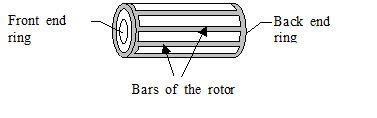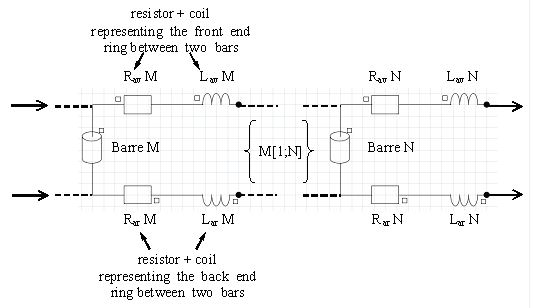If N is the number of the bars of the squirrel cage, the electrical circuit contains N solid conductors, 2N resistors and 2N coils.

## Squirrel cage: the two types of the electrical circuit closing

The electrical circuit of a squirrel cage can be closed in two ways, depending on the machine representation mode: modeling of a part (fraction) of the machine (1/4, 1/2, …) or of the entire machine.

Thus, the closing type of an electrical circuit is linked to the boundary conditions assigned to the frontiers of the correspondent finite element domain.

• cycling closing if the entire motor is described or if the considered part is physically symmetric to the neighboring parts (an even number of the poles are represented in the finite element domain),
• anticyclic closing if the described part of the motor is physically antisymmetric to the neighboring parts (an odd number of poles represented).

The two types of the electrical circuit closing are represented in the figure below.

• Cyclic closing: entire motor, symmetry (even number of poles)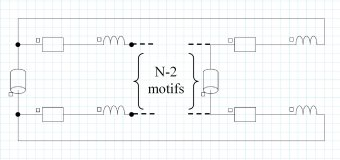=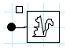• Anticyclic closing: antisymmetry (odd number of poles pairs)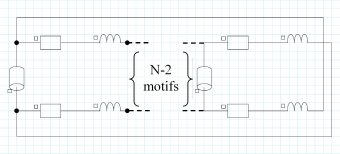=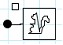## Characteristics of the squirrel cage macro component

The squirrel cage macro component is defined by four parameters:

• the number of bars N, present in the fraction of the modeled motor (finite element domain)
• the resistance R of the end ring part between two adjacent bars
• the inductance L of the end ring part between two adjacent bars